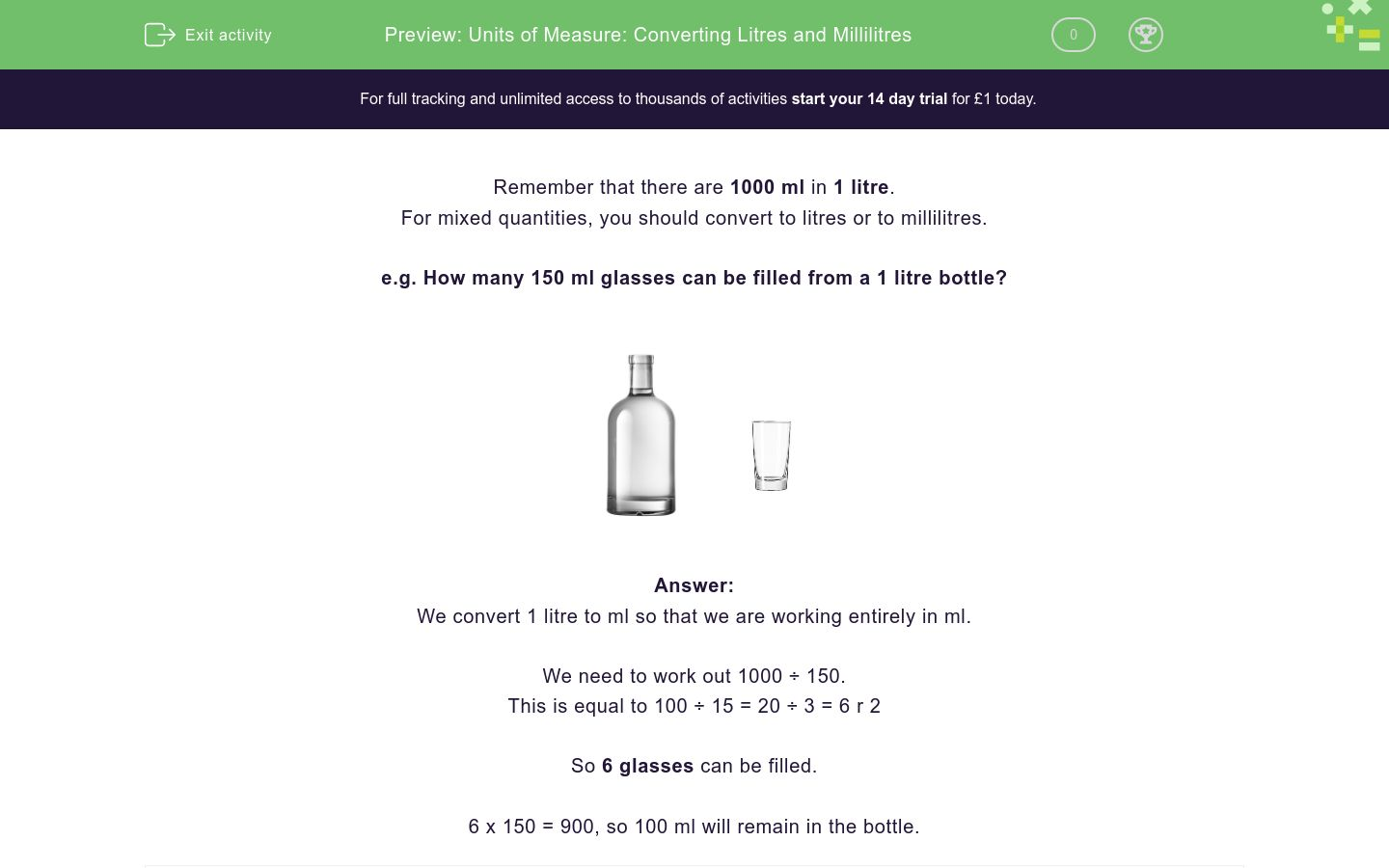# Units of Measure: Converting Litres and Millilitres

In this worksheet, students answer problems based on capacity.Key stage:  KS 2

Curriculum topic:   Measurement

Curriculum subtopic:   Convert Between Metric Measurements

Difficulty level:### QUESTION 1 of 10

Remember that there are 1000 ml in 1 litre.

For mixed quantities, you should convert to litres or to millilitres.

e.g. How many 150 ml glasses can be filled from a 1 litre bottle?We convert 1 litre to ml so that we are working entirely in ml.

We need to work out 1000 ÷ 150.

This is equal to 100 ÷ 15 = 20 ÷ 3 = 6 r 2

So 6 glasses can be filled.

6 x 150 = 900, so 100 ml will remain in the bottle.

How many 250 ml glasses can be filled from a 1 litre bottle?

How many 200 ml glasses can be filled from a 1½ litre bottle?

Adam is told that he should drink 3 litres of water per day.

How many 200 ml glasses is this?

Anne is told that she should drink 2½ litres of water per day.

How many 200 ml glasses is this?

15

12½

10½

Ben is told to take a 5 ml teaspoon of cough mixture four times a day.

For how many days will a 100 ml bottle last?

A bottle of lemonade holds 2½ litres.

Sue pours out 7 glasses holding 330 ml each.

How many ml remain in the bottle?

Jack drinks 2.5 litres of water a day.

Jill drinks 1/5 more.

How many millilitres does Jill drink?

A tomato plant needs 8.4 l of water per week.

How many millilitres is this per day?

A bucket contains 20 litres of water.

How many 2500 ml bottles are required to fill the bucket?

A watering can contains 12 litres of water.

How many millilitres will it hold when it is 1/5 full?

• Question 1

How many 250 ml glasses can be filled from a 1 litre bottle?

4
EDDIE SAYS
1 litre x 1000 = 1000 ml 1000 ml ÷ 250 ml = 4. So 4 glasses can be filled.
• Question 2

How many 200 ml glasses can be filled from a 1½ litre bottle?

7
EDDIE SAYS
1 1/2 litres = 1.5 x 1000 = 1500 ml 1500 ml ÷ 200 ml = 7 1/2. So 7 glasses can be filled.
• Question 3

Adam is told that he should drink 3 litres of water per day.

How many 200 ml glasses is this?

15
EDDIE SAYS
3 litres x 1000 = 3000 ml 3000 ml ÷ 200 ml = 15 Adam should drink 15 200 ml glasses a day.
• Question 4

Anne is told that she should drink 2½ litres of water per day.

How many 200 ml glasses is this?

12½
EDDIE SAYS
2 1/2 litre x 1000 = 2500 ml 2500 ml ÷ 200 ml = 12 1/2. Anne should drink 12 1/2 200ml glasses a day.
• Question 5

Ben is told to take a 5 ml teaspoon of cough mixture four times a day.

For how many days will a 100 ml bottle last?

5
EDDIE SAYS
5 ml x 4 times a day = 20. 100ml ÷ 20 = 5. The bottle will last 5 days.
• Question 6

A bottle of lemonade holds 2½ litres.

Sue pours out 7 glasses holding 330 ml each.

How many ml remain in the bottle?

190
EDDIE SAYS
2 1/2 litre x 1000 = 2500 ml 2500 ÷ 330 = 7 rem 190 ml There is 190 ml left in the bottle.
• Question 7

Jack drinks 2.5 litres of water a day.

Jill drinks 1/5 more.

How many millilitres does Jill drink?

3000
EDDIE SAYS
2.5 litres x 1000 = 2500 ml Find 1/5 of 2500 = 2500 divided by 5 = 500 ml. Jill drinks 2500 ml + 500 ml = 3000 ml.
• Question 8

A tomato plant needs 8.4 l of water per week.

How many millilitres is this per day?

1200
EDDIE SAYS
8.4 liters x 1000 = 8400 ml per week 8400 divided by 7 days in a week = 1200 ml per day
• Question 9

A bucket contains 20 litres of water.

How many 2500 ml bottles are required to fill the bucket?

8
EDDIE SAYS
20 litres x 1000 = 20000 ml 20000 ml divided by 2500 ml = 8 8 bottles are required to fill the bucket.
• Question 10

A watering can contains 12 litres of water.

How many millilitres will it hold when it is 1/5 full?

2400
EDDIE SAYS
12 litres x 1000 = 12000ml 1/5 of 12000 = 12000 divided by 5 = 2400 It will hold 2400 ml when it's 1/5 full.
---- OR ----